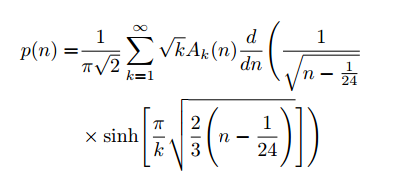This error appears when you have written a \left command, without a corresponding \right command. The \left and \right commands are used for dynamically resizing parenthesis. The delimiters allowed which can be used with \left and \right commands are () [] | \| \{ \} or the dot .. If you do not use the \left and \right commands when placing parentheses around large items such as fractions and integrals, the parenthesis will not be the same size as the expression contained: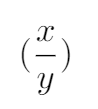You can place fitted brackets around a fraction by writing \left( \frac{x}{y} \right). This will create nicer looking parenthesis which are the same size as the expression contained within them: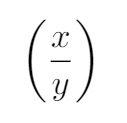If you write a \left command, but do not finish the expression with a \right command, you will generate the error shown below:

main.tex, line 5

Missing \right. inserted.

<inserted text> \right . l.5 $\left(x=\frac{1}{2}$ I've inserted something that you may have forgotten. (See the <inserted text> above.) With luck, this will get me unwedged. But if you really didn't forget anything, try typing 2' now; then my insertion and my current dilemma will both disappear. [1

# Common Causes

Forgetting to include a \right:

The most basic cause of this error occurs when you have written a \left command but have forgotten to include a corresponding \right as shown below:

$\pi = \left( \int_{-\infty}^{+\infty} e^{-x^2} dx )$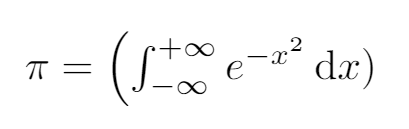This will generate an error as we have written a \left at the start of our expression, but we have not written a \right at the end of it. The correct way to write this expression is:

$\pi = \left( \int_{-\infty}^{+\infty} e^{-x^2} dx \right)$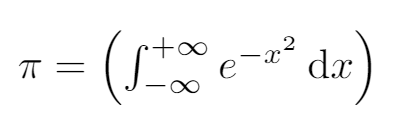If you would like to only have a \left delimiter, with no \right, you can solve this by including a \right.. The dot indicates that this \right will be blank.

Using \left and \right commands around alignment characters:

Another common mistake is when \left and \right commands are used around alignment characters as shown below:


\begin{align}
f(x) =
\left( 1 &+ 2x \right)\\
+ \left( 3x^2 &+ 4x^3 \right)
\end{align}

This is not allowed, and will cause an error to be generated. The correct way to write this is to use \bigl and \bigr commands as shown below:

\begin{align}
f(x) =
\bigl( 1 &+ 2x \bigr)\\
+ \Bigl( 3x^2 &+ 4x^3 \Bigr)
\end{align}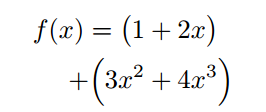These \bigl and \bigr commands are not dynamically fitted, and are a set size. The size will need to be chosen by hand, however they pose the advantage of not needing to be paired in the same way as \left and \right commands. The choices of sizing -- in order of increasing size -- are \big, \Big, \bigg, and \Bigg. If you use these statements in pairs, such as \biggl[ and \biggr], LaTeX will perform some syntax checking for you.

Using \left for piecewise functions:

A common cause of error occurs when writing piecewise functions such as the one shown below: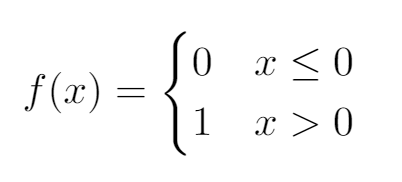If you write this as:

\[
f(x)= \left\{
\begin{array}{lr}
0 & x\leq 0 \\
1 & x > 0
\end{array}
/]

you will generate an error, as there is no \right. One way around this would be to include a blank \right by writing \right. as shown below:

\[
f(x)= \left\{
\begin{array}{lr}
0 & x\leq 0 \\
1 & x > 0
\end{array}
\right.
/]

A better way to write this is to avoid \left and \right commands entirely by using the cases enviromnent provided by the amsmath environment as shown below:

% In your preamble
\usepackage{amsmath}

% In the main body of your document
\[
f(x)= \begin{cases}
0 & x\leq 0 \\
1 & x > 0
\end{cases}
/]

Using line breaks inside \left and \right commands:

When writing long expressions, you sometimes may want to include line breaks as shown below:

The number of ways of writing a number $n$ as a sum of positive integers is given by

\begin{align*}
p(n)=
&\frac{1}{\pi \sqrt{2}} \sum_{k=1}^\infty \sqrt{k} A_k(n)
\frac{d}{dn} \left({\frac {1} {\sqrt{n-\frac{1}{24}}} \\
&\times \sinh \left[ {\frac{\pi}{k}
\sqrt{\frac{2}{3}\left(n-\frac{1}{24}\right)}}\right]
}\right)
\end{align*}

This will generate an error, as we are not allowed to include a line break \\ inside \left and \right commands. The correct way to write this expression is to once again use \Biggl and \Biggr commands as shown below:

The number of ways of writing a number $n$ as a sum of positive integers is given by

\begin{align*}
p(n)=
&\frac{1}{\pi \sqrt{2}} \sum_{k=1}^\infty \sqrt{k} A_k(n)
\frac{d}{dn} \Biggl({\frac {1} {\sqrt{n-\frac{1}{24}}} \\
&\times \sinh \Biggl[ {\frac{\pi}{k}
\sqrt{\frac{2}{3}\Biggl(n-\frac{1}{24}\Biggr)}}\Biggr]
}\Biggr)
\end{align*}`